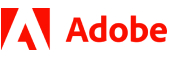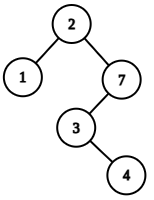New update is available. Click here to update.
Topics

# Kth Largest Element in BST

Moderate0/80+1 more companies

## Problem statement

Given the root node of a Binary Search Tree (BST), you have to return the Kth largest element in the BST.

For Example:
``````If K is 4 and the tree is depicted by the following image then,
````````````The 4th largest element in the given BST is 1. So the output will be 1.
``````
Follow-up :
`````` Try to do it in O(1) space without using recursion.
``````
Detailed explanation ( Input/output format, Notes, Images )
Constraints :
``````1 <= T <= 100
1 <= N <= 5 * 10^3
1 <= K <= N
0 <= X <= 10^9

Where ‘X’ is the value at the node and ‘N’ is the no. of nodes in given BST.

Time Limit: 1 sec.
``````
Sample Input 1 :
``````2
2 1 4 -1 -1 3 -1 -1 -1
4
2 1 7 -1 -1 3 -1 -1 4 -1 -1
3
``````
Sample Output 1 :
``````1
3
``````
Explanation For Sample Input 1 :
``````For First Case - Same as explained in above example.

For the second case -
K is 3 and the tree is depicted by the following image then,
````````````The 3rd largest element in the given BST is 3. So the output will be 3.
``````
Sample Input 2 :
``````2
4 3 -1 2 -1 1 -1 -1 -1
1
3 1 8 -1 2 -1 -1 -1 -1
3
``````
Sample Output 2 :
``````4
2
``````Console